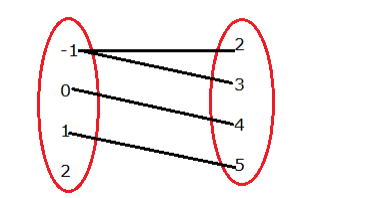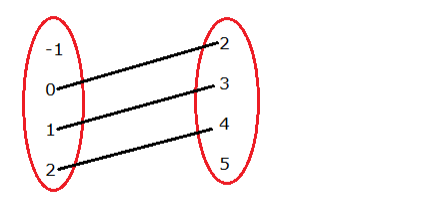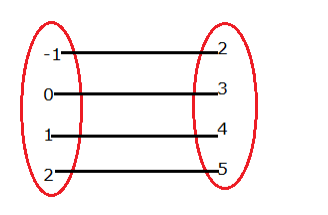# Prove that

Question:

Let $A=\{-1,0,1,2\}$ and $B=\{2,3,4,5\}$. Find which of the following are function from A to B. Give reason.

(i) $f=\{(-1,2),(-1,3),(0,4), 1,5)\}$

(ii) $g=\{(0,2),(1,3),(2,4)\}$

(iii) $h=\{(-1,2),(0,3),(1,4),(2,5)\}$

Solution:

(i) Given: $A=\{-1,0,1,2\}$ and $B=\{2,3,4,5\}$

Function:

(i) all elements of the first set are associated with the elements of the second set.

(ii) An element of the first set has a unique image in the second set.

$f=\{(-1,2),(-1,3),(0,4),(1,5)\}$$f=\{(-1), 2),(-1), 3),(0,4),(1,5)\}$

Here, -1 is coming twice.

Hence, it does not have a unique (one) image.

∴ f is not a function

(ii) Given: $A=\{-1,0,1,2\}$ and $B=\{2,3,4,5\}$

Function:

(i) all elements of first set is associated with the elements of second set.

(ii) An element of first set has a unique image in second set.

$g=\{(0,2),(1,3),(2,4)\}$Here, -1 is not associated with any element of set B

Hence, it does not satisfy the condition of the function

∴ g is not a function.

(iii) Given: $A=\{-1,0,1,2\}$ and $B=\{2,3,4,5\}$

Function:

(i) all elements of first set is associated with the elements of second set.

(ii) An element of first set has a unique image in second set.

$h=\{(-1,2),(0,3),(1,4),(2,5)\}$Here, (i) all elements of set A are associated to element in set B.

(ii) an element of set A is associated to a unique element in set B

∴ h is a function.